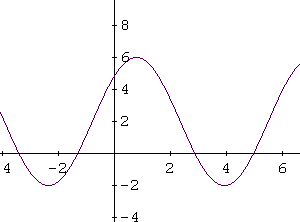# Tutorial on Sine Functions (2)- Problems

This is a tutorial on the relationship between the amplitude, the vertical shift and the maximum and minimum of the sine function.

## Problem 1

Function F is a sine function given by
F(x) = a*sin(bx + c) + d

with a > 0.
1. Show that the maximum value Fmax and the minimum value Fmin of F(x) are given by
Fmax = d + a
Fmin = d - a
2. Show that d = (Fmax + Fmin) / 2
3. Show that a = (Fmax - Fmin) / 2

Solution to Problem 1:

1. We start by using the fact that
-1 <= sin(bx + c) <= 1
2. Multiply all terms of the above double inequality by a
-a <= sin(bx + c) <= a
3. Add d to all terms of the double inequality above
d - a <= sin(bx + c) + d <= d + a
4. sin(bx + c) + d is the expression that define F(x), hence
d - a <= F(x) <= d + a
5. F(x) has a minimum value Fmin and a maximum value Fmax given by
Fmin = d - a
Fmax = d + a
6. Add the left terms and the right terms of
Fmax = d + a and Fmin = d - a to obtain

Fmax + Fmin = 2d
7. Divide both sides of the above by 2 to obtain
d = (Fmax + Fmin) / 2
8. Add the left terms and the right terms of
Fmax = d + a and -Fmin = -d + a to obtain

Fmax - Fmin = 2a
9. Divide both sides by 2 to obtain
a = (Fmax - Fmin) / 2

## Problem 2

The graph of the sine function F given by
F(x) = a*sin(x) + d

with a > 0, is shown below.
Use the graph and the results of problem 1 above to find a and d.Solution to Problem 2:

1. From the graph, the maximum value Fmax = 6 and the minimum value Fmin = -2, hence
d = (Fmax + Fmin) / 2 = 2
and
a = (Fmax - Fmin) / 2 = 4